@sticky-add: @:libyli @:title-slide /no-status no-libyli # // comment- {{ nd.vars.author }} - - Talk at {{ nd.vars.venue }}, {{ nd.vars.date }} # $whoami @:#overview overview no-libyli ## Overview - Introduction - Imbalanced classification problems - The Problem (and performance measures) - Reweight, resampling, etc - Correcting k-NN (*$\gamma$-NN@:accro*) - Focusing on the F-Measure optimization (*CONE@:accro*) - Interpretability - Pleading for interpretability - Adversarial Input↔Parameter Regularization (*AI↔PR@:accro*) - Previous, current and future approaches - Discussion @eval-header:$o.s = 2 @eval-header: return highlightLi($o.s, 1) # @copy: overview ## Imbalanced Problems: Examples // generally, but at the lab - Anomaly detection *// incl images* - unsafe situations in videos // bluecime, first step into it for me - defect detection in images // often as out-of-distribution though - anormal heart beat in ECG - Fraud detection - fraudulent checks - credit card fraud (physical, online) - financial fraud (French DGFIP) // Dir. Géné. des Fin. Pub. - @:displaynone // TODO: add illustr ## Imbalanced Classification Problems // generally, but at the lab- Binary classification -$+$positive class: minority class, anomaly, rare event, … {.no} -$-$negative class: majority class, normality, typical event, … {.no} - Confusion matrix (of a model vs a ground truth) - TP: true positive - FP: false positive - TN: true negative - FN: false negative - Some measures {.padli .densemath} - Precision:$prec=\frac{TP}{TP+FP}$- Recall:$rec=\frac{TP}{P} = \frac{TP}{TP+FN}$-$F_\beta$-measure:$F_\beta = (1+\beta^2)\frac{prec\cdot rec}{\beta^2 \cdot prec + rec}$\ *(higher is better) {.dense} ## F-measure vs Accuracy ? -$F_\beta = (1+\beta^2)\frac{prec\cdot rec}{\beta^2 \cdot prec + rec} = \frac{(1+\beta^2)\cdot (P - FN)}{1 + \beta^2 P - FN + FP}$\ {.no} -$accuracy = \frac{TP + TN}{P+N} = 1 - \frac{FN+FP}{P+N}${.no} - Accuracy inadequacy (e.g.$N=10000, P=10$) - lazy "all$-$" classifier ($TP=0, TN=N, FP=0, FN=P$) -$\textstyle \textcolor{orange}{accuracy} = \frac{0 + N}{P + N} = \frac{10000}{10100} \textcolor{orange}{= 99\\%}$-$\textstyle \textcolor{orange}{F_\beta} = \frac{(1+\beta^2) (P - P)}{1 + \beta^2 P - P + 0} \textcolor{orange}{= 0}$-$F_\beta$-measure challenges - discrete (like the accuracy) - non-convex (even with continuous surrogates) - **non-separable**, i.e.$F_\beta \ne \sum_{(x_i, y_i) \in S}...$// TODO maybe a schema for the trivial case ## Ok, but I'm doing gradient descent, so …- Gradient:$0.2$⇒$-7.21$,$0.5$⇒$-2.89$,$0.8$⇒$-1.80$,$1$⇒$-1.44$- Example, gradient intensity is the same for: -$10+$wrongly classified with an output proba. of$0.2$-$40-$correctly classified with an output proba$0.8$- i.e., lazily predicting systematically$0.2$(for$+$) yields a "stable" solution with$10+$vs$40-$@:info-slide # Disclaimer: multiclass$F_\beta$-measure**s** // todo might style... @eval-header: return highlightLi($o.s, 2) # @copy: overview ## Counteracting Imbalance - Undersampling the majority class $-$ - Oversampling class $+$ - Generating fake $+$ - Using a weighted-classifiers learner // For models that learn, can reweigh but not perfect (ideal ratio depends on Bayesian error and régularisation etc) can x valid? @eval-header: return highlightLi($o.s, 3) # @copy: overview @:paperattrib #gammann no-libyli ## A Corrected Nearest Neighbor Algorithm Maximizing the F-Measure from Imbalanced Data - **Rémi Viola**, Rémi Emonet , Amaury Habrard, **Guillaume Metzler**, Sébastien Riou, Marc Sebban - ??? ## k-NN:$k$Nearest Neighbor Classification- k-NN {.step} - to classify a new point - find the closest k points (in the training section) - use a voting scheme to affect a class - efficient algorithms (K-D Tree, Ball Tree) - Does k-NN still matter? *// yes non-conv thing, easy adaptability, etc* - non-linear by design (with similarity to RBF-kernel SVM) - no learning, easy to patch a model (add/remove points) // e.g. ECML - Limits of k-NN for imbalanced data? ## Limits of k-NN for imbalanced data? 1. k-NN behavior in uncertain areas - i.e., for some feature vector, the class can be$+$or$-$- i.e., the Bayes Risk is non zero - ✔ not so bad (respects imbalance) 2. k-NN behavior around boundaries - i.e., what happens if classes are separate but imbalanced - ✖ sampling effects cause problems ## k-NN at a boundary (1000$+$)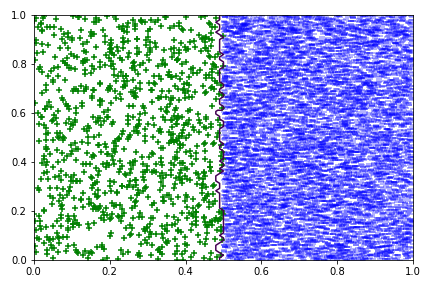## k-NN at a boundary (100$+$)## k-NN at a boundary (10$+$)## k-NN: increasing k? // (from 1 to 11)# @copy: gammann ##$\gamma$-NN Idea: push the decision boundary- Goal: correct for problems due to sampling with imbalance - Genesis: GAN to generate "$+$" around existing ones - ⇒ unstable, failing, complex @:no - Approach - artificially make$+$closer to new points - how? by using a different distance for$+$and$-$- the base distance to$+$gets multiplied by a parameter$\gamma$(intuitively$\gamma \le 1$if$+$is rare) \def{\x}{\mathbb{x}} d_\gamma(\x,\x_i) = \begin{cases} d(\x,\x_i) & \text{if} \; \x_i\in S_-,\\ \gamma \cdot d(\x,\x_i) & \text{if} \;\x_i\in S_+. \end{cases} ##$\gamma$-NN: varying$\gamma$with two points##$\gamma$-NN: varying$\gamma$with a few$+$-$\gamma$-NN can control how close to the$-$s it pushes the boundary ##$\gamma$-NN: Algorithm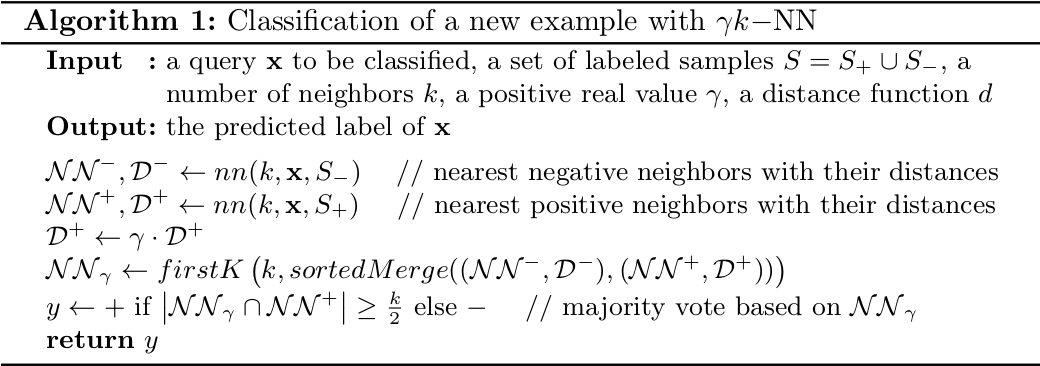- Trivial to implement - Same complexity as k-NN (at most twice) - Training - none, as k-NN -$\gamma$is selected by cross-validation (on the measure of interest) ##$\gamma$-NN: a way to reweight distributions - In uncertain regions // when we have more and more points - At the boundaries // depends on intrisic dimensionality @:/no-status ## Results on public datasets (F-measure)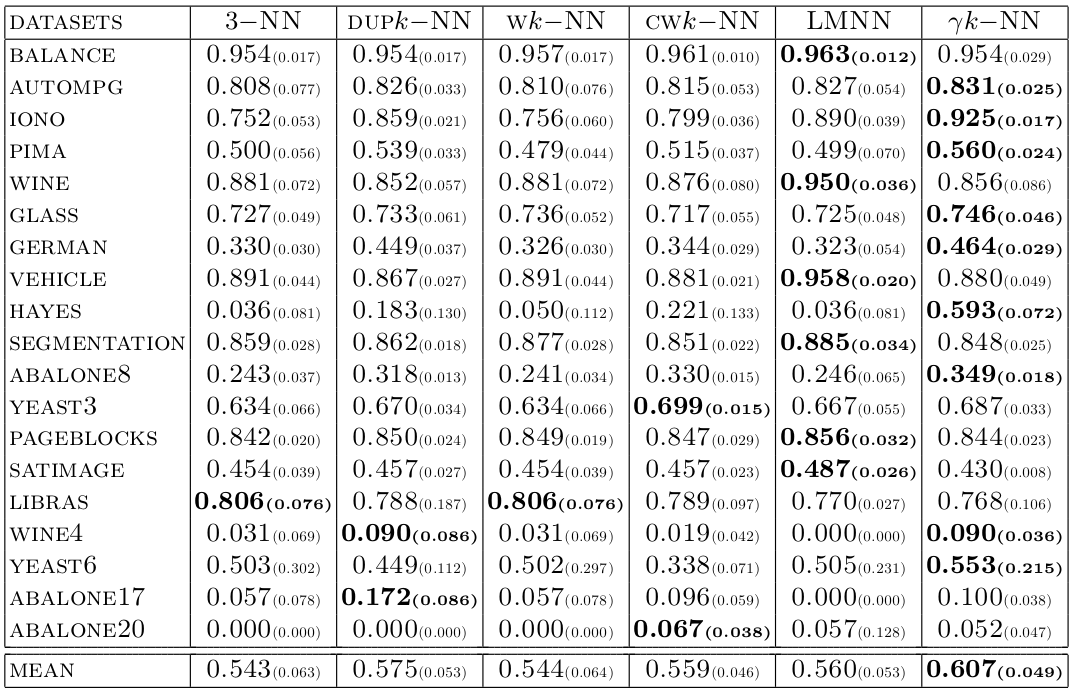@:/no-status ## Results on DGFiP datasets (F-measure) // underline = second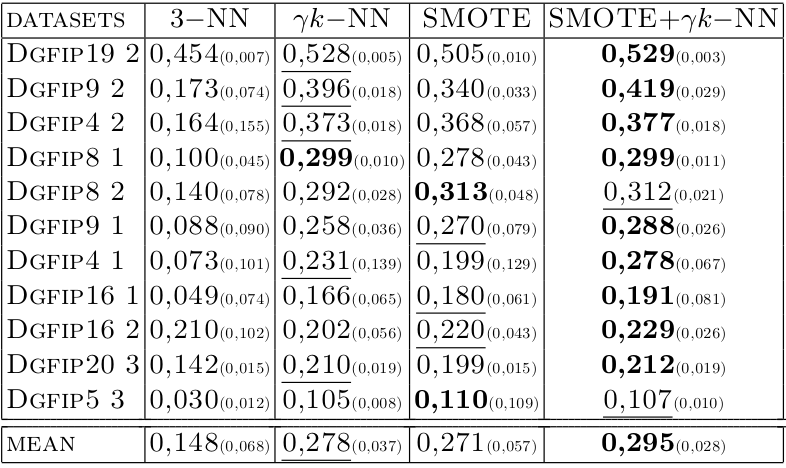@eval-header: return highlightLi($o.s, 4) # @copy: overview @:paperattrib no-libyli ## From Cost-Sensitive Classification to Tight F-measure Bounds - **Kevin Bascol**, Rémi Emonet, Elisa Fromont, Amaury Habrard,
**Guillaume Metzler**, Marc Sebban    - AISTATS2019 ## Optimizing the $F_\beta$-measure? - Reminder {.padli .densemath} - Precision: $prec=\frac{TP}{TP+FP}$ - Recall: $rec=\frac{TP}{P} = \frac{TP}{TP+FN}$ - $F_\beta$-measure: $F_\beta = (1+\beta^2)\frac{prec\cdot rec}{\beta^2 \cdot prec + rec}$ - **Non-separability**, i.e.    $F_\beta \ne \sum_{(x_i, y_i) \in S}...$
*NB: accuracy is separable, $acc = \sum_{(x_i, y_i) \in S} \frac{1}{m} \delta(y_i - \hat{y_i})$ @:denser* - ⇒ The loss for one point depends on the others {.no} - ⇒ Impossible to optimize directly {.no} - ⇒ Impossible to optimize on a subset (minibatch) {.no} ## Weighted classification for $F_\beta$ - $F_\beta = \frac{(1+\beta^2)\cdot (P - FN)}{1 + \beta^2 P - FN + FP} = \frac{(1+\beta^2)\cdot (P - e_1)}{1 + \beta^2 P - e_1 + e_2}$

{.no} - The $F_\beta$-measure is linear fractional *(in $e = (e_1, e_2) = (FN, FP)$) @:dense* - i.e. $F_\beta = \frac{\langle a', e\rangle + b}{\langle c, e\rangle + d} = \frac{A}{B}$ {.no} - Relation to weighted classification - $\hphantom{\Leftrightarrow } F_\beta \ge t$      (we achieve a good, above $t$, $F_\beta$ value) {.no} - $\Leftrightarrow A \ge t\cdot B$ {.no} - $\Leftrightarrow A - t\cdot B \ge 0$ {.no} - $\Leftrightarrow (1+\beta^2)\cdot (P - e_1) - t ( 1 + \beta^2 P - e_1 + e_2) \ge 0$ {.no} - $\Leftrightarrow (- 1 - \beta^2 + t) e_1 - t e_2 \ge - P (1 + \beta^2) + t ( 1 + \beta^2 P)$ {.no} - $\Leftrightarrow (1 + \beta^2 - t) e_1 + t e_2 \le - P (1 + \beta^2) + t ( 1 + \beta^2 P)$ {.no} - ⇒ so, we can minimize the weighted problem
with class weights $a(t) = (1 + \beta^2 - t, t)$ {.no}
- TODO derive down to the existing weight and corr. weighted classif - can we have guarantees on the f we obtained (as we don't know how to opt it) - there exist a weighted classification pb that given the optimal f (talk about the fractional linear thing and v?) - this weight (tstar) is unknown
@:no-libyli ## Inspiration for our work - **title:** *Optimizing F-measures by cost-sensitive classification* - **by:** Parambath, S. P., Usunier, N., and Grandvalet, Y. - **in:** NIPS 2014 -  {.no} - Main result (reformulation) {.step} - given a value of $t$ that is at most at $\frac{\varepsilon_0}{2}$ from the optimal $t^\star$ - given a weighted-classification learner - then the optimal $F^\star$ is not too far from the observed $F$ - They conclude that grid search on $t$ is sound
i.e., we'll get arbitrary close to the optimal $F_\beta$-measure
*(and they experiment with 19 $t$ values) @:dense* {.step} ## Geometric interpretation of bounds - A reinterpretation of the bound // (and/or its derivations) - given - a value of $t$ (and class weights $a(t)$), and - a weighted-classifier $h$ (learned with weight $a(t)$) - … yielding an error profile $e$ and a correponding $F_\beta(e)$ - **then**\*, for any $t'$ (that would yield a classifier $e'$), - *(if $t'$ is close to $t'$ then $F(e')$ is close to $F(e)$) @:dense* - $F(e')$ is upper bounded by an expression linear in $|t - t'|$ // cannot go higher\* All bounds are up to $\varepsilon_1$, the sub-optimality of the learned weighted classifier @:footnote ## Visualizing the bound of Parambath et al.## Deriving a tighter bound - Same objective: drawing cones - New, tighter, asymmetric bounds - Given - all definitions - NB: $\varepsilon_1$ the sub-optimality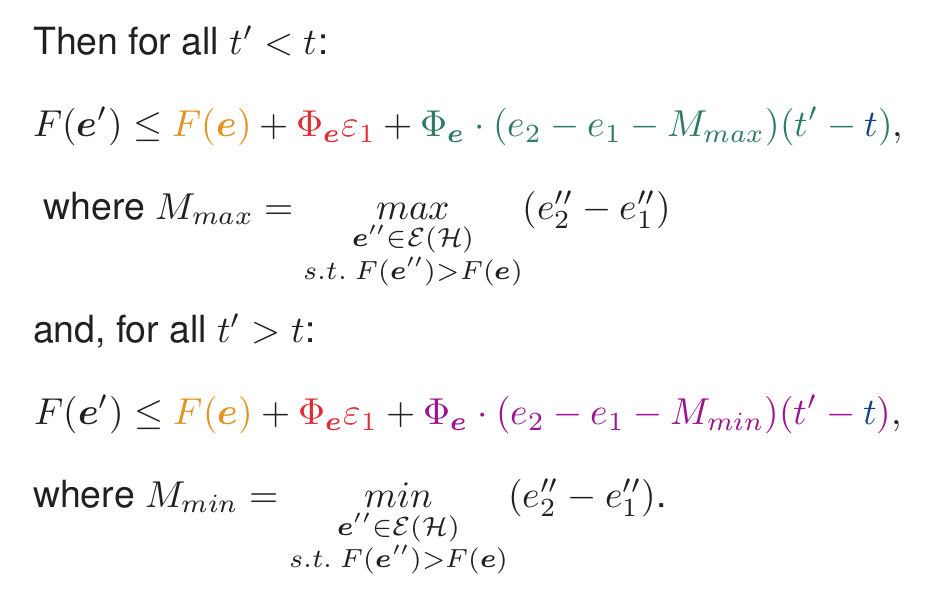## Visualizing the bound of Parambath et al.## Visualizing our bound## Using the bound to better explore $t$ values## CONE: example resultsNon-vacuous bounds, incremental algo., early increase. ## CONE: numerical results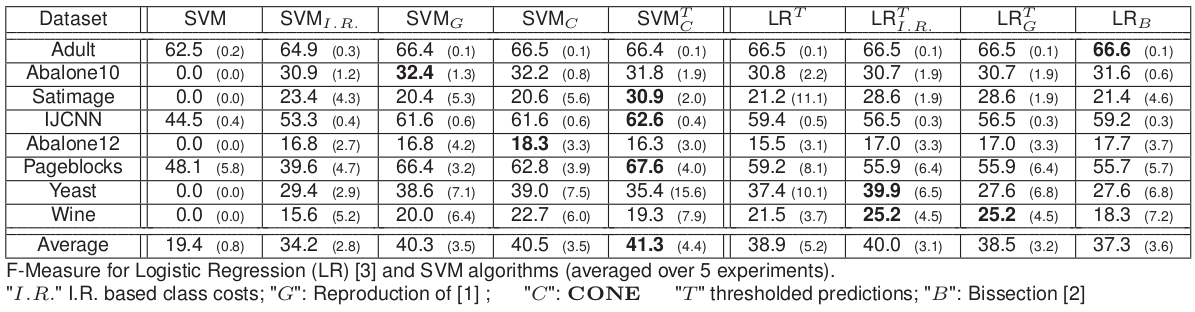No huge gain, better $F$-measure in average. ## CONE: Perpsectives - We - provided a geometric interpretation as cones - derived new, tighter bounds - proposed a search algorithm using the bounds - Work in progress - bounds in generalization - lower bounds@eval-header: return highlightLi(3, 1) # @copy: overview ## Interpretability - Why, what ## Example of interpretable patterns - We overlay on the series the pattern that were most relevant to the decision taken by the classifier- What side (left or right) would be more convincing? ## Approaches towards interpretability - Black box models - Intepretability by design - constraints from the model structure @eval-header: return highlightLi(3, 2) # @copy: overview @:paperattrib no-libyli ## Learning Interpretable Shapelets for Time Series Classification through Adversarial Regularization - **Yichang Wang**, Rémi Emonet, Elisa Fromont, Simon Malinowski, **Etienne Menager, Loic Mosser**, Romain Tavenard - ??? + arxiv2019 @:/no-status ## Adversarial Input↔Parameter Regularization (*AI↔PR@:accro*) {.dense}## Optimal Transport, Duality and Adversarial Learning -
L_d(\theta_d) = \mathop{\mathbb{E}}_{\tilde{x}\sim\mathbb{P}_{S}} \left[ D(\tilde{x}) \right] - \mathop{\mathbb{E}}_{x\sim\mathbb{P}_{x}} \left[D(x)\right] + \lambda \mathop{\mathbb{E}}_{\hat{x}\sim\mathbb{P}_{\hat{x}}}\left[(||\nabla_{\hat{x}}{D(\hat{x})}||_2-1)^2\right]
- Gradient penalty - Interpolation to cover the space## 3 Losses for 3 Phases - Classifier training
\;\;\;\;L_C(\theta_c) = ...
- Discriminator Training, using fixed shapelets
\;\;\;\;L_d(\theta_d) = \mathop{\mathbb{E}}_{\tilde{x}\sim\mathbb{P}_{S}} \left[ D(\tilde{x}) \right] - \mathop{\mathbb{E}}_{x\sim\mathbb{P}_{x}} \left[D(x)\right] + \lambda \mathop{\mathbb{E}}_{\hat{x}\sim\mathbb{P}_{\hat{x}}}\left[(||\nabla_{\hat{x}}{D(\hat{x})}||_2-1)^2\right]
- Shapelet regularization, using the fixed discriminator
\;\;\;\;L_r(\theta_s) = - \mathop{\mathbb{E}}_{ {\tilde{x}}\sim\mathbb{P}_{S}}[D(\tilde{x})]
@:/no-status ## Algorithm## Discriminator work across iterations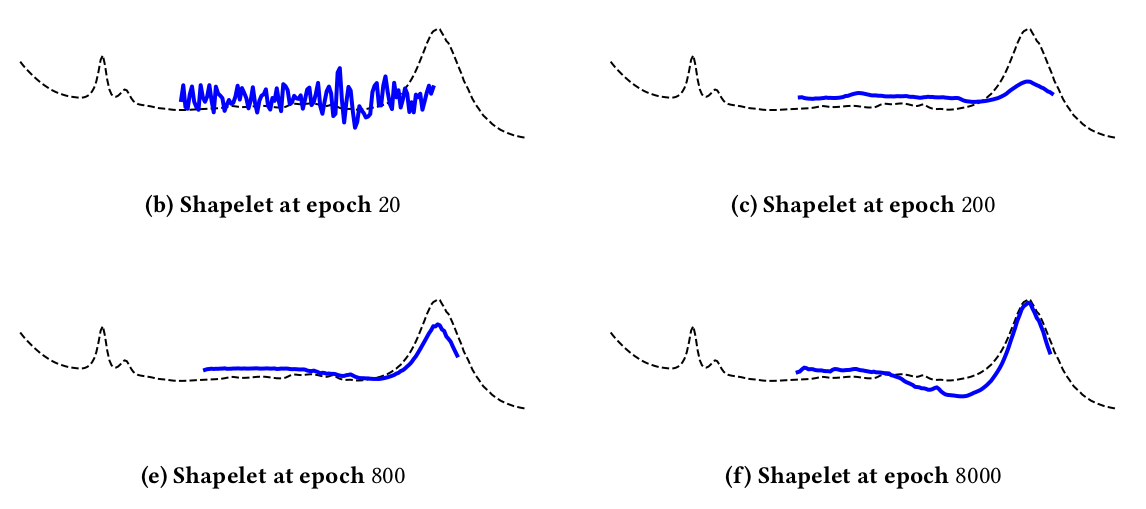## Results on 85 datasets (vs Fast Shapelets)## Results *(vs Learning Shapelets, non-interpretable)@:dense*@eval-header: return highlightLi(3, 3) # @copy: overview ## More... - Perspectives around *AI↔PR@:accro* - multivariate - group-lasso used well - deeper regularization - Other interpretable families of methods - probablisistic models - auto-encoders with regularizations and constraints - neural EM etc @eval-header: return highlightLi(4, 1) # @copy: overview @:/title-slide # Thank you! Questions?